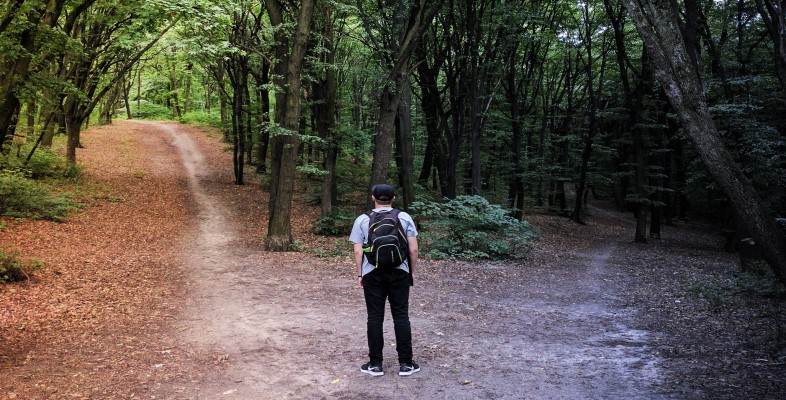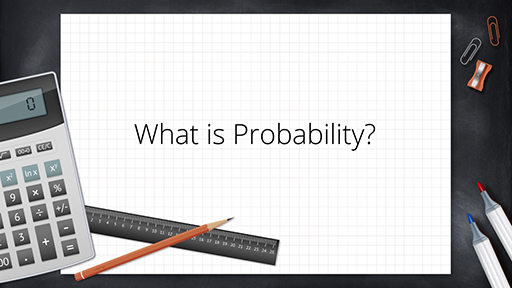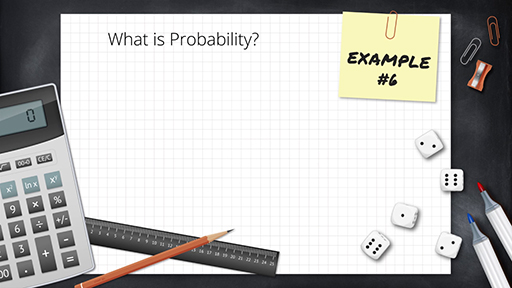Decision trees and dealing with uncertainty

Start this free course now. Just create an account and sign in. Enrol and complete the course for a free statement of participation or digital badge if available.

Free course

1 Dealing with uncertainty: an introduction to probability

Allow approximately 1 hour 30 minutes to complete this section.

Decision-making is often undertaken in an uncertain world. That is, you might have some doubts about the reliability of the data you have been provided with, as well as the future environment in which you will be operating. In business, uncertainty regarding the future can cover a very wide range of scenarios, including:

• political – who will be running the country in five years’ time? What will be their view on, for example, tax, regulation, etc.?
• economic – will the country be in recession, or booming? What will interest rates be?
• market – how will your product market develop? Will there be new entrants, leavers or substitutes?

As decision-makers, you need to find strategies to deal with uncertainty and so, in this section, you will be introduced to the topic of probability.

A knowledge of probability enables you to assess the likelihood of something happening. For example, what is the chance that a 55-year-old person will buy a new car in the next 12 months?

You might subdivide the data – what is the probability that a 55-year-old man will buy a car within the next 12 months?

You can then go further by attaching conditions. For example, what is the probability that a 55-year-old man, who bought his last car three years ago, will buy a new car within the next 12 months?

Or even, if a 55-year-old man has purchased a new car within the last three years, how long will it be before he buys a new car?

Unfortunately, probability theory is quite a complex subject and so in this course you will only consider the most basic ideas. These ought to suffice for the kinds of decisions that managers need to make most of the time. In the next activity you will be introduced to those basic ideas.

Activity 1 What is probability?

Timing: Allow approximately 20 minutes to complete this activity

In Videos 1 and 2, you will be introduced to the basic ideas of probability. An understanding of probability allows you to quantify uncertainty.

You will build on your understanding of probability, especially later on in this course when you learn about decision trees. So you may need to watch Videos 1 and 2 a few times until you feel comfortable with how probability starts to address the question of how to deal with uncertainty in decision-making.

Part 1

Watch Video 1. You may like to make notes in the text box below.Skip transcript: Video 1 An introduction to probability: part 1

Transcript: Video 1 An introduction to probability: part 1

NARRATOR:
What is probability? Probability gives us a way of measuring or assessing uncertainty. It measures the likelihood of certain random events. We're going to go through some examples.
Take a 6-sided dice. What's the probability that we'll throw a 3? We know that there are 6 possible outcomes: 1, 2, 3, 4, 5, and 6. But there's only one 3. And so there's a 1 in 6 chance, or a 1 in 6 probability, of rolling number 3. We would express that probability as 1 divided by 6, which is 0.167.
Taking the same 6-sided dice, what's the probability that we'll throw an even number? Well, there's 3 even numbers on a dice: 2, 4, and 6, and there's 6 possible outcomes, so we'd express this as 3 over 6, which is a half, or 0.5.
What's the probability that we'll throw an even number greater than 3? There are 2 even numbers greater than 3: 4 and 6. So there's 2 numbers and 6 possible outcomes. So the probability here is 2 out of 6, which is one third, or 0.33. We can see that probability compares the number of times the desired outcome happens with the total number of possible outcomes.
What's the probability that you'll throw an odd number greater than 3? The answer here is 1 in 6 because there's only 1 odd number greater than 3 - that's 5. And so we say there's a 1 out of 6 probability.
What's the probability that we'll throw a number greater than 3? Well, there are 3 numbers greater than 3: 4, 5, and 6. So we would immediately think it's 3 out of 6, which is 0.5.
But another way of doing this is to say, what's the probability of throwing even numbers greater than 3, and adding that to the probability of throwing odd numbers greater than 3. And so the probability of throwing a number greater than 3 should equal the probability of throwing the even numbers plus the probability of throwing the odd numbers.
Here, we've got the probability of throwing an even number greater than 3 as 0.333 and the probability of throwing an odd number greater than 3 as 0.167. If you add them together, it's 0.5. This only works because they're what we call mutually exclusive events. A number greater than 3 cannot be both odd and even.
So each of the numbers: 4, 5, and 6 can only be an even number or an odd number. And when you have mutually exclusive events, you can do this adding up calculation. So one way of calculating the probability of an outcome is to compare the number of ways that outcome may occur with the total number of possible outcomes.
End transcript: Video 1 An introduction to probability: part 1
Video 1 An introduction to probability: part 1
Interactive feature not available in single page view (see it in standard view).
To use this interactive functionality a free OU account is required. Sign in or register.
Interactive feature not available in single page view (see it in standard view).

Part 2

Watch Video 2. You may like to make notes in the text box below.Skip transcript: Video 2 An introduction to probability: part 2

Transcript: Video 2 An introduction to probability: part 2

NARRATOR:
Let's throw 2 6-sided die. What's the probability that you'll throw a total equal to 7? Remember what we need to do. We're going to compare the number of times it could happen with the total number of complete possibilities.
Here's all the complete possibilities. There are 36 ways that the two die could fall. Out of these, how many ways are there of getting 7? I've put them all in red. There's 6 possible ways or 6 combinations. So 6 over 36, which is the same as one sixth, which is 0.167.
What's the probability of throwing first a 2 and then an even number greater than 3? Well, let's start with the first die. Throwing a 2, we know happens 1 out of 6 times. There's only one 2, and there's 6 outcomes. This means that anything which comes after, whatever that probability, is only going to happen one sixth of the time. So it's going to be whatever this probability is times one sixth.
The second throw, throwing an even number greater than 3, is going to happen 2 out of 6 times. But the first row is only happening one sixth of the time. Therefore, the second throw can only happen one sixth of its normal probability. And so we multiply two sixths, which is actually one third, by one sixth to get two thirty-sixths, or one eighteenth. So events that follow each other can be multiplied by the individual probabilities.
Here's some overall rules about probability. The probability of all possible outcomes has to equal 1. What's the probability of throwing an odd number? 3 over 6. What's the probability of throwing an even number? 3 out of 6. In other words, the probability of everything happening is 1.
For combined mutually exclusive events, we can add probabilities. We looked at mutually exclusive events in example 5. Combined events - one dice, then another - can be found by multiplying their probabilities. And of course, if something doesn't have a possibility of it happening, we can still use the rule of how often it happens compared to the total. So if the number of times it happens is 0, then the probability must be 0.
And finally, before we leave the intrinsic approach, we can also use history, studies, or market research to come up with probabilities. Here's something from a medical piece of research. 2619 men were found to have certain risk factors to do with coronary heart disease. Of those men, at the end of the 8-year study, 246 of them had suffered with heart disease.
So if the total possible men that could have coronary heart disease with a risk factor is 2619 but only 246 went on to actually get it, then the probability of getting it is this figure here. The number of those who did over the total number who could have. So 246 over 2619. In other words, a probability of 0.094.
End transcript: Video 2 An introduction to probability: part 2
Video 2 An introduction to probability: part 2
Interactive feature not available in single page view (see it in standard view).
To use this interactive functionality a free OU account is required. Sign in or register.
Interactive feature not available in single page view (see it in standard view).

Discussion

From Video 2 you learnt that probability is a way of measuring uncertainty. The probability of an event may be taken as the proportion of times that an event can occur out of the total possible events. The video provided some simple examples of how you might estimate a probability.

Most people will have an instinctive feel for what probability is. Probability is a measure of how likely it is that something will occur. How might you assess that likelihood?

Probability: first principles

To begin, you might be able to derive a number from first principles.

For example, it is hopefully obvious that if someone throws a ‘normal’ six-sided die, the number ‘3’ is likely to land face up 1 in 6 times. You might say that the probability of throwing a ‘3’ is , or one sixth.

Of course, if someone throws a die six times then they might get ‘3’ more than once or even no ‘3’s. It is only if the die is thrown thousands of times that the person will notice that roughly one sixth of the throws result in a ‘3’ landing face up.

Probability: considering past behaviour

Another way of arriving at a probability might be to observe past behaviour. For example, the Eurovision song contest has, as of April 2019, had 66 winners (some years there have been joint winners). The Republic of Ireland has won it seven times. So you might conclude that the Republic of Ireland has a probability of winning the next contest. Coming to such a conclusion is, of course, rather simplistic. You would need to consider how much past success indicates the chance of future success, given that the performers are usually different.

Probability: subjective opinion

One last way you might assign a probability is through simply expressing a subjective opinion. For example, if you watch the Eurovision performers prior to voting, you might arrive at a view of which act is most likely to appeal to the voters (nowadays, a combination of the public and appointed judges). Of course, this probability might be very different to that assigned by another watcher.

The concept of probability could also be used to consider how the gambling industry functions and the interplay between odds and subjective probabilities, but this will not be covered in this course.

In business scenarios, it is not often that you can rely on the first approach of deriving a number from first principles of probability only. More often, you have to arrive at a probability based on a combination of some data (via a market survey, for example) and experience.

Before proceeding, the definition and mathematical rules for probability need to be explained. From the die example earlier, a reasonable definition of a probability can be concluded as follows:

•
• Probability is expressed as a ratio whose value is positive. You cannot have negative probabilities.

• A probability is less than or equal to 1. This means that a probability cannot exceed 1.

• The total of all probability outcomes must equal 1.

For example, if the probability of something happening is 0.45, the probability of it not happening must be .

In the next section you will have the opportunity to check your understanding of probability.

B874_1